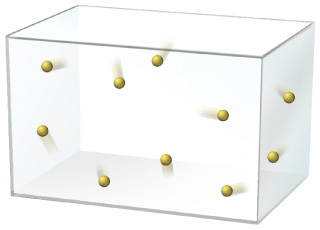# Problem: The following picture represents a sample of gas at a pressure of 1 atm, a volume of 1 L, and a temperature of 25 oC. What would happen to the pressure if the volume were reduced to 0.5 L and the temperature increased to 260 oC?

###### FREE Expert Solution

Combined gas law:

$\overline{)\frac{{\mathbf{P}}_{\mathbf{1}}{\mathbf{V}}_{\mathbf{1}}}{{\mathbf{T}}_{\mathbf{1}}}{\mathbf{=}}\frac{{\mathbf{P}}_{\mathbf{2}}{\mathbf{V}}_{\mathbf{2}}}{{\mathbf{T}}_{\mathbf{2}}}}$

The given values are:

P1 = 1 atm
P2 = ??
V1 = 1 L
V2 = 0.5 L
T1 = 25˚C + 273.15 = 298.15 K
T2 = 260˚C + 273.15 = 533.15 K

We can rearrange the combined gas law to solve for P2:

98% (119 ratings)###### Problem Details

The following picture represents a sample of gas at a pressure of 1 atm, a volume of 1 L, and a temperature of 25 oCWhat would happen to the pressure if the volume were reduced to 0.5 L and the temperature increased to 260 oC?

Frequently Asked Questions

What scientific concept do you need to know in order to solve this problem?

Our tutors have indicated that to solve this problem you will need to apply the Chemistry Gas Laws: Combined Gas Law concept. You can view video lessons to learn Chemistry Gas Laws: Combined Gas Law. Or if you need more Chemistry Gas Laws: Combined Gas Law practice, you can also practice Chemistry Gas Laws: Combined Gas Law practice problems.